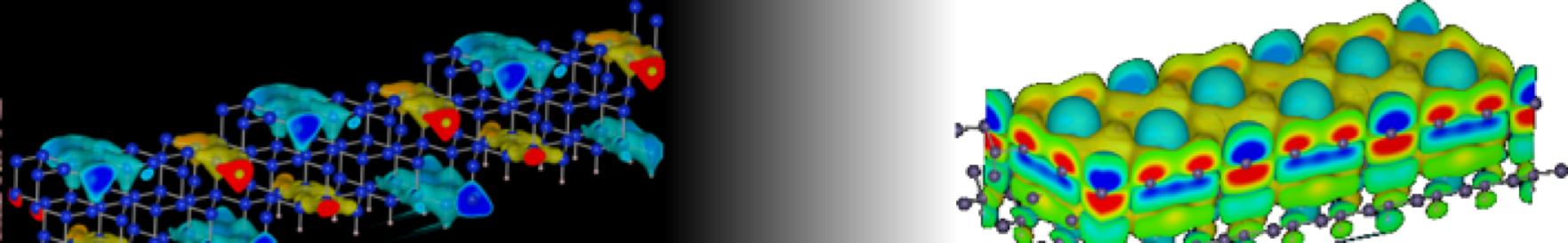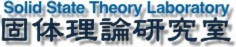SST Lab Dokuwiki Header@surface

サイドバー

SST Lab Dokuwiki Headerseminar:graphene

総合演習

グラフェンを例に、より詳しい計算の演習を行う。

プログラムの概要

a0 = 2.461 # Lattice constant of Graphene

from math import sqrt
c1 = ( a0*sqrt(3.0)/2.0, a0/2.0, 0.0)
c2 = (-a0*sqrt(3.0)/2.0, a0/2.0, 0.0)
c3 = (0.0, 0.0, a0*8.0)              # Height of 3D unit cell

from ase import Atom
atom1 = Atom('C', (1.0/3.0, 2.0/3.0, 0.5))
atom2 = Atom('C', (2.0/3.0, 1.0/3.0, 0.5))

from ase import Atoms
box1 = Atoms([atom1, atom2], pbc = True)
box1.set_cell([c1, c2, c3], scale_atoms = True)

from ase.calculators.jacapo import Jacapo
solver1 = Jacapo(nbands = 8, kpts = (2, 2, 1), pw = 150, dw = 150)
box1.set_calculator(solver1)
solver1.calculate()

単位格子の設定

b0 = 1.42 # C-C bond length

from math import sqrt
a0 = b0*sqrt(3.0)
ax = a0*sqrt(3.0)/2.0    # ax = b0*3.0/2.0
ay = a0/2.0
az = a0*8                # Height of 3D unit cell
c1 = ( ax, ay, 0.0)
c2 = (-ax, ay, 0.0)
c3 = (0.0, 0.0, az)

unit_cell = [c1, c2, c3] # Unit cell

原子の位置と種類

v1 = (1.0/3.0, 2.0/3.0, 0.5)
v2 = (2.0/3.0, 1.0/3.0, 0.5)
positions = [v1, v2]

from ase import Atom

list_of_atoms = []
for pos in positions:
atm = Atom('C', pos)
list_of_atoms.append(atm)

# # list_of_atoms is a list of [atom1, atom2]

計算パラメーターの設定

# -------------------------
# Calculational conditions
# -------------------------
kwargs = {}
kwargs['nbands'] = 8        # Number of Valence electrons
kwargs['kpts'] = (2, 2, 1)  # k-point sampling in the 1st BZ
kwargs['pw'] = 150          # Energy cutoff for wave functions
kwargs['dw'] = 150          # Energy cutoff for charge density

計算の実行

# ---------------------------
# Common part of calculations
# ---------------------------
from ase import Atoms
box1 = Atoms(list_of_atoms, pbc = True)
box1.set_cell(unit_cell, scale_atoms = True)
from ase.calculators.jacapo import Jacapo
solver1 = Jacapo(**kwargs)
box1.set_calculator(solver1)
solver1.calculate()

結果の出力

# ------------------
# Output on screen
# ------------------
print box1.get_total_energy()
seminar/graphene.txt · 最終更新: 2019/12/05 09:20 by kimi

ページ用ツール